• 基本版本的kmeans算法的实现，附带wine数据集，并做了可视化
• kmeans算法代码 Kmeans算法基本思想是：首先给出聚类的个数K，然后初始随机给定K个待聚类中心（也叫簇中心），按照最邻近原则把待分类样本点分到各个类，也就是样本点到哪个簇中心的距离最近，这个样本点就属于哪一...
• ## Kmeans算法

热门讨论 2012-10-17 21:34:53
经典的Kmeans算法 资料非常详细，包括word文档，PPT，matlab code
• ## KMeans算法

千次阅读 2019-04-28 20:28:14
KMeans算法 from sklearn.datasets import make_blobs import matplotlib.pyplot as plt from sklearn.cluster import KMeans from sklearn.metrics import silhouette_score from sklearn.metrics import ...
KMeans算法
from sklearn.datasets import make_blobs
import matplotlib.pyplot as plt
from sklearn.cluster import KMeans
from sklearn.metrics import silhouette_score
from sklearn.metrics import silhouette_samples

#自己创建数据集
X,y = make_blobs(n_samples=500, n_features=2, centers=4, random_state=1)
#n_samples:样本点数，n_features:每个点的特征，random_state:随机性，设置为1方便教学数据，centers:数据中心，后续可以发现分4个簇时反映簇内和簇间差异的轮廓系数最好
#这里生成的数据是自带标签y的，而聚类算法并不需要标签;
print(X.shape) #(500,2)

#将得到的数据集按照X的特征进行可视化
fig, ax1 = plt.subplots(1) #建立子图，1表示只建立一个，返回fig画布，ax1一个子图对象
ax1.scatter(X[:,0], X[:,1], marker='o', s=8) #marker:点的形状，s:点的尺寸
plt.show()

##由于生成数据自带标签，我们可以根据标签对这个数据集进行可视化
#color = ['red','pink','orange','gray']
#fig, ax1 = plt.subplots(1)
#
#for i in range(4):
#    ax1.scatter(X[y==i,0], X[y==i,1], marker='o', s=8, c=color[i])
#
#plt.show()
n_cluster = 3
cluster = KMeans(n_clusters=n_cluster, random_state=0).fit(X) #在不知道类别的情况下暂定为3
y_pred = cluster.labels_ #聚类后数据的标签
print(y_pred)

#KMeans因为并不需要建立模型或者预测结果，因此我们只需要fit就能得到聚类结果了
#KMeans也有接口predict和fit_predict，表示学习数据X并对X的类进行预测
#但所得到的的结果和我们不调用predict，直接fit之后属性的labels一模一样
pre = cluster.fit_predict(X)
print(pre==y_pred)

#重要属性cluster_centers_，查看质心
centroid = cluster.cluster_centers_
print(centroid)
print(centroid.shape)

#重要属性inertia，查看总距离平方和
inertia = cluster.inertia_
print(inertia)

#将聚类后的数据集进行可视化
color = ['red','pink','orange','gray']
fig, ax1 = plt.subplots(1)

for i in range(n_cluster):
ax1.scatter(X[y_pred==i,0], X[y_pred==i,1], marker='o', s=8, c=color[i])

ax1.scatter(centroid[:,0], centroid[:,1],marker='x', s=14, c='black')
plt.show()

#聚类算法的衡量指标----轮廓系数
'''
前面cluster.inertia_表示所有点到质心的距离平方和，会发现质心越多(分的簇越多)，值越小，并不能很好地评价聚类效果；
依赖于簇内稠密程度(簇内差异小)和簇间离散程度(簇外差异大)来评价聚类效果，轮廓系数是常用的聚类算法的评价指标；
样本与其自身所在的簇中的其他样本的相似度a，等于样本与同一簇中所有其他点之间的平均距离；
样本与其他簇中的样本的相似度b，等于样本与下一个最近的簇中得所有点之间的平均距离；
轮廓系数：S=(b-a)/max(a,b) 值位于-1~1，且值大于0说明聚类还行，越接近1效果越好；
'''
s1 = silhouette_score(X, y_pred) #返回的是一个数据集中，所有样本的轮廓系数的均值
s2 = silhouette_samples(X, y_pred) #参数与轮廓系数一致，但返回的是数据集中每个样本自己的轮廓系数
print(s1)
print(s2)

根据轮廓系数来衡量聚类算法
from sklearn.cluster import KMeans
from sklearn.datasets import make_blobs
from sklearn.metrics import silhouette_samples, silhouette_score
import matplotlib.pyplot as plt
import matplotlib.cm as cm
import numpy as np

X,y = make_blobs(n_samples=500, n_features=2, centers=4, random_state=1)
for n_clusters in [2,3,4,5,6,7]:
n_clusters = n_clusters
fig, (ax1, ax2) = plt.subplots(1, 2) #一个画布上同时生成两个图
fig.set_size_inches(15, 7) #画布的尺寸
ax1.set_xlim([-0.1, 1]) #第一个图的横坐标范围，显示的是每个数据的轮廓系数
ax1.set_ylim([0, X.shape + (n_clusters + 1) * 10]) #第一个图的纵坐标范围，显示的是数据量，每类数据间间隔为10
clusterer = KMeans(n_clusters=n_clusters, random_state=10).fit(X)
cluster_labels = clusterer.labels_
silhouette_avg = silhouette_score(X, cluster_labels) #所有数据的轮廓系数的平均值
print("For n_clusters =", n_clusters,
"The average silhouette_score is :", silhouette_avg)
sample_silhouette_values = silhouette_samples(X, cluster_labels)
y_lower = 10
for i in range(n_clusters):
ith_cluster_silhouette_values = sample_silhouette_values[cluster_labels == i] #提取第i类数据的轮廓系数
ith_cluster_silhouette_values.sort() #对轮廓系数进行从小到大的排序；从小到大排列只需要sort(reverse=True)
size_cluster_i = ith_cluster_silhouette_values.shape #每一类数据的个数
y_upper = y_lower + size_cluster_i #每一类数据在y轴上的分布上限
color = cm.nipy_spectral(float(i)/n_clusters) #每一类的显示颜色

ax1.fill_betweenx(np.arange(y_lower, y_upper)
,ith_cluster_silhouette_values
,facecolor=color
,alpha=0.7
)
ax1.text(-0.05
, y_lower + 0.5 * size_cluster_i
, str(i))
y_lower = y_upper + 10
ax1.set_title("The silhouette plot for the various clusters.")
ax1.set_xlabel("The silhouette coefficient values")
ax1.set_ylabel("Cluster label")
ax1.axvline(x=silhouette_avg, color="red", linestyle="--") #参考线
ax1.set_yticks([])
ax1.set_xticks([-0.1, 0, 0.2, 0.4, 0.6, 0.8, 1])
colors = cm.nipy_spectral(cluster_labels.astype(float) / n_clusters)
ax2.scatter(X[:, 0], X[:, 1]
,marker='o' #点的形状
,s=8 #点的大小
,c=colors
)
centers = clusterer.cluster_centers_
ax2.scatter(centers[:, 0], centers[:, 1], marker='x',
c="red", alpha=1, s=200)
ax2.set_title("The visualization of the clustered data.")
ax2.set_xlabel("Feature space for the 1st feature")
ax2.set_ylabel("Feature space for the 2nd feature")
plt.suptitle(("Silhouette analysis for KMeans clustering on sample data "
"with n_clusters = %d" % n_clusters),
fontsize=14, fontweight='bold')
plt.show()




展开全文• 概述：KMEANS算法又被成为K均值算法，是一种常用的聚类算法，由于不需要根据给定的训练集训练模型因此是一种无监督学习算法。其本质是根据选定的参数K将数据分类成K类，在聚类过程中从单一样本开始通过不断计算聚类...概述：KMEANS算法又被成为K均值算法，是一种常用的聚类算法，由于不需要根据给定的训练集训练模型因此是一种无监督学习算法。其本质是根据选定的参数K将数据分类成K类，在聚类过程中从单一样本开始通过不断计算聚类数据的均值来作为整个类的中心进而再将距离此类别中心最近的数据纳入同一类。算法原理：1、以下图样本散点图展示数据集的整体分布情况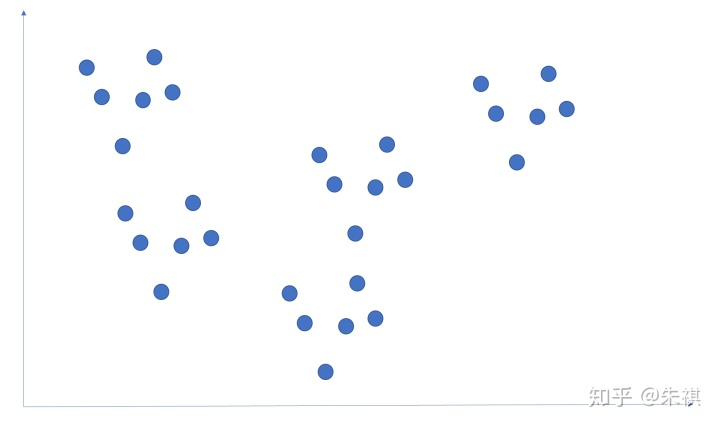2、K值是KMEANS最重要的选择参数，直接决定着数据聚类的类别数量，在选择K值后，会在数据中随机选择K个数据样本最为初始中心点，如K=3，则结果如下图所示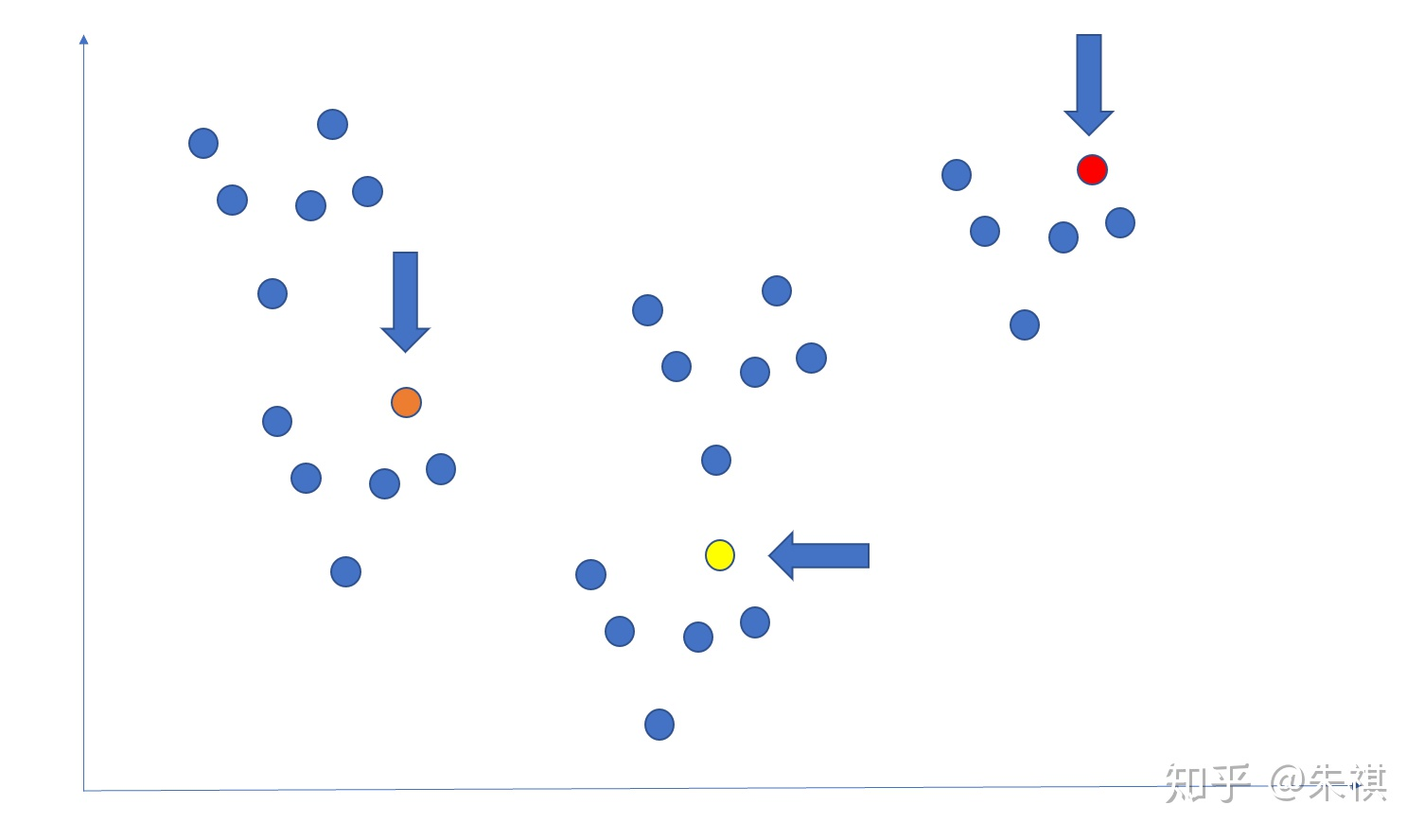3、计算和中心点距离最近的点，将其归入同类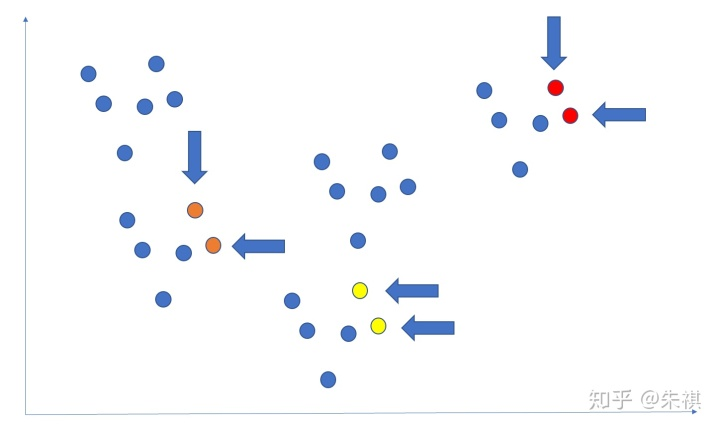4、每个类别当有了两个以上的数据时，类的中心就会发生变化，因此类中一旦有新的数据被划入时就需要重新计算整个类的中心点，这一步的计算也是整个算法的核心，所以称为K均值算法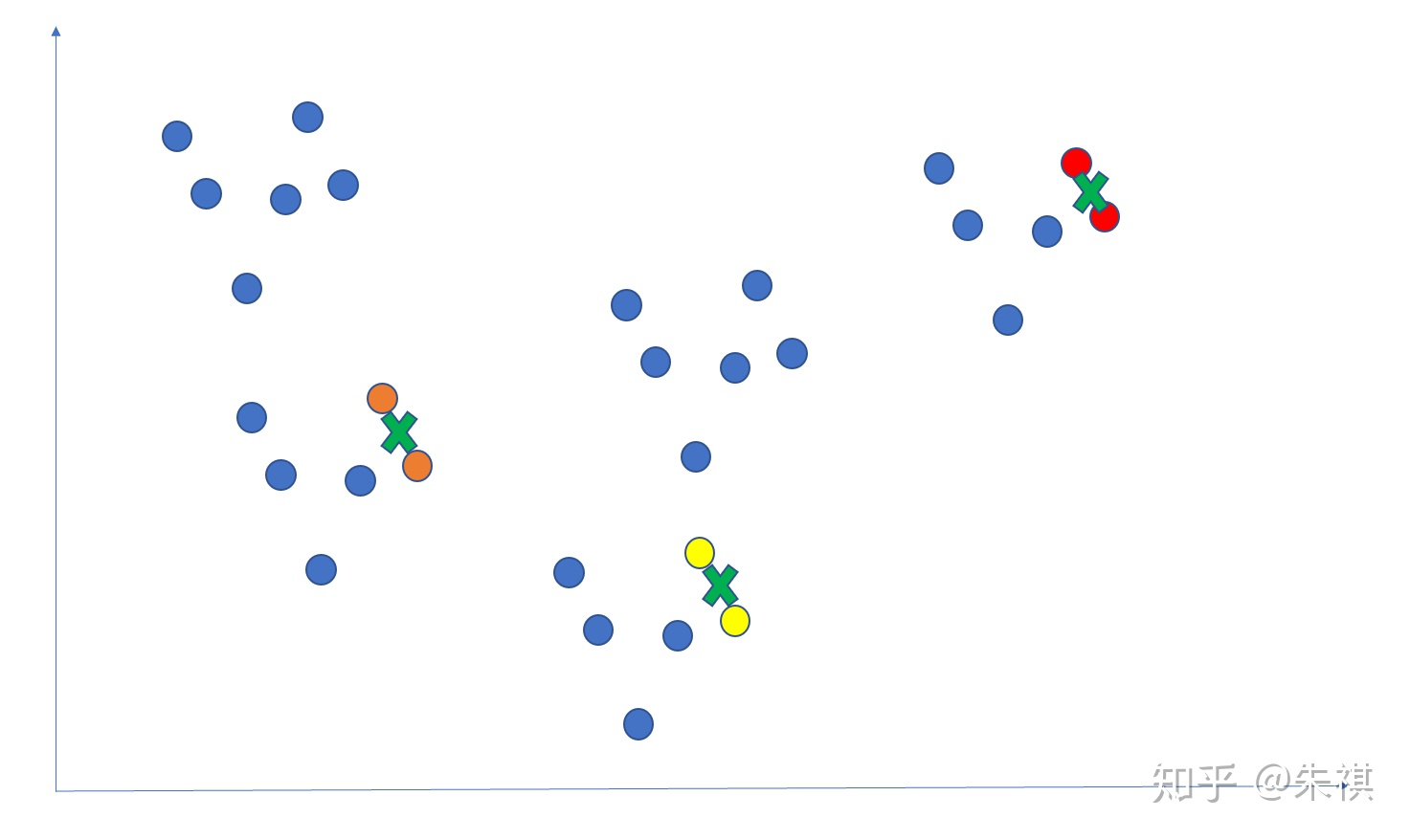5、通过几步计算之后的结果，能够更直观的展示出类的聚合情况和中心点的位置情况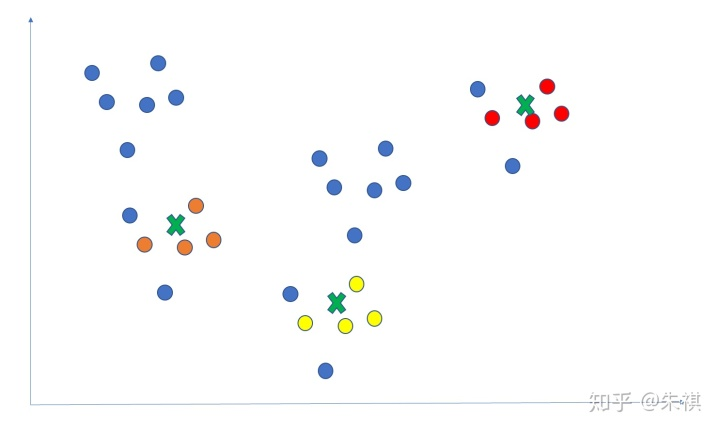6、判断聚类过程结束的标准有两个，一是中心点的位置不再发生变化，即结果收敛；二是执行了最够多次的迭代次数（通俗可以理解为计算了几次中心点位置）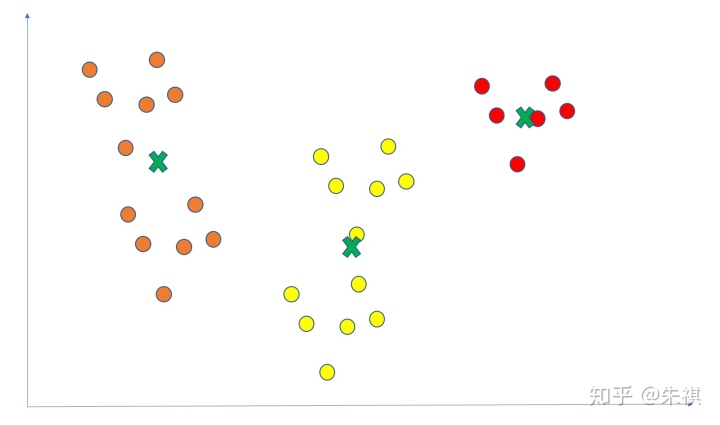注意事项：1、K值是整个算法中最重要的参数，但是也是最不好确定的参数，如果需要比较好的确定K值，需要采用其他验证算法，如计算样本离最近聚类中心的总和，总和越小，则聚类的效果越好；轮廓系数，轮廓系数的范围为-1至1之间，数字越大则聚类效果越好；兰德指数，范围为-1至1之间，数字越大则聚类效果越好；同质化得分，如果所有的聚类都只包含属于单个类的成员的数据点则聚类结果将满足同质性，其取值范围为0至1之间，值越大意味着聚类结果与真实情况越吻合。2、以上验证方法虽然对于确定K值有效，但是验证过程需要额外的计算力资源，并且占用的计算力接近于聚类过程所需要的计算力资源，数据集如果较大，则计算力的消耗会产生叠加效应。3、较为简易的方法为，从数据集中随机抽取部分小规模数据，以散点图等可视化手段来观察数据的可能聚类数量，以此来判断K的取值。这种方法可以认为是经验法的一种表现形式，相比经验法的完全定性分析，随机抽取数据观察能够在经验的基础上增加定量的分析部分，虽然随机抽取的数据也可能有误差，但是抽取的数据量越多，则准确度越高。4、因为初始的中心点选择是根据K的值随机选择K个点，所以选择的随机性加上迭代过程造成算法的结果只是局部最优解，毕竟反复的计算最短距离的点和类的中心都是在局部已经聚合的类的基础上进行的，而不是从全局的范围进行。算法使用场景：1、隐含类别的数据较为平衡的情况，如隐含类别的数据量差别较大，则聚类的效果就较差。2、数据最好是凸数据，即隐含类别间的差异越大，则聚类效果越好，因为中心点不再变化所需要的迭代次数较少，比较容易收敛。3、一般作为数据预处理，或者用于辅助分类贴标签使用，因为在已经经过分类的数据上再进行聚类，准确度会非常高。
展开全文• 其实Kmeans在机器学习中是一种无监督学习算法，整个算法很好理解， 算法第一步: 在数据集中随机选择K个质点作为质心， 第二步： 计算整个数据集中每个元素到K个质心的距离，选择最近的质心，将该元素划分到该 ...
其实Kmeans在机器学习中是一种无监督学习算法，整个算法很好理解，

算法第一步:     在数据集中随机选择K个质点作为质心，

第二步：  计算整个数据集中每个元素到K个质心的距离，选择最近的质心，将该元素划分到该 质心

第三步:   经过第二步计算，每个质心都有一个集合，重新计算质心:计算每个集合的均值。

重复第二步和第三步: 理想的状态的，第三步重新计算的质心和原质心一样不变，说明已经达到理想状态，可以停止算法了，而实际上，我们通常给一个最大计算次数。

该算法弊端:

1. 很容易受到离群点数据的影响

2. K值很难选择

kmeans函数:

class sklearn.cluster.KMeans(n_clusters=8, init=’k-means++’,
n_init=10, max_iter=300, tol=0.0001,
precompute_distances=’auto’, verbose=0,
random_state=None, copy_x=True,
n_jobs=None, algorithm=’auto’)

例子:

import numpy as np
from sklean.cluster import KMeans
X = np.array([
[1,2,3],
[4,0,9],
[1,4,6],
[10,2,3],
[10,3,0],
[10,9,1]
])
km = KMeans(n_cluster=2).fit(X)
print km.labels_         #label
y = km.predict([[1,4,5]])  #预测
print y
print km.cluster_centers_  #质心


展开全文...